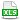HomeTechniques and Tips@RISK Simulation: Numerical ResultsSum of All Iteration Values

# 6.19. Sum of All Iteration Values

Applies to: @RISK for Excel 4.x–7.x

I see a RiskMean( ) function, but no RiskSum( ). I would like a sum that totals all of the values that occur in a given cell during a simulation. Is this possible within the framework of @RISK?

Response:
The accompanying workbook shows three methods.:

1. The simplest, Method 1, multiplies the simulated mean by number of iterations obtained from the RiskSimulationInfo( ) function available in @RISK 6.0 and later.

2. For earlier versions of @RISK, you can use Method 2. It's the same technique, but with a helper cell to calculate the number of iterations.

3. You can also use Method 3. Insert all the data in the worksheet, using the technique in Placing Iteration Data in Worksheet with RiskData( ), then sum the data. This method has the advantage of being a little more transparent, but it uses a lot more space in your workbook, and it fails if you change the number of iterations to more than the number you originally planned.

Last edited: 2015-06-30

•SumOfAllIterations.xls